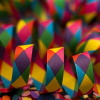#### You may also like### Rationals Between...

What fractions can you find between the square roots of 65 and 67?### Repetitiously

Can you express every recurring decimal as a fraction?### The Square Hole

If the yellow equilateral triangle is taken as the unit for area, what size is the hole ?

# Equal Equilateral Triangles

### Why do this problem?

The bisected equilateral triangle (30-60-90) is an important shape for students to become familiar with, and the early questions in this problem draw out the compound forms built from this triangle.
The final question, to which the other questions serve as an introduction, explores surd forms and the circumstances when one length can, or cannot, be a rational multiple of another.

### Possible approach

Preceding these questions with 'playtime' using cut out triangles to form patterns may be a very useful preliminary for many students.

### Key questions

• While looking at the screen shot on the Hints page : are the green and yellow hexagons really the same size or just close ? How can we be sure of that? [this draws out the relationship between the yellow and green triangles, including that they have equal area - draw attention to the line of symmetry for each shape to help students visualise this relationship]
• Still looking at the screen shot on the Hints page : can the larger hexagon be made from green triangles ? If so make it, or if not explain why this cannot be done.

### Possible extension

The second of the key questions above is challenging, able students should be encouraged to reason this thoroughly.
The following problems make a natural follow on from this activity: The Square Hole and Impossible Triangles?

### Possible support

There are a number of activities which can provide valuable auxiliary experiences for students working on this problem :

• Drawing first the equilateral triangle using only a straight edge and compasses, and then creating the isosceles triangle, likewise, will give a strong sense for the symmetry of each triangle and the relationship between them.
• For students who are not able to make any progress with grasping the last question, 'Can you make an equilateral triangle from yellow triangles that is the same size as an equilateral triangle made from green triangles ?' the earlier questions, although a lead up to this, nevertheless make a very good activity in themselves. Some children 'play' a long time with cut out triangles arranged on a table, motivated by the aesthetic appeal of emerging pattern possibilities, this is excellent grounding for other mathematical ideas to be built on.